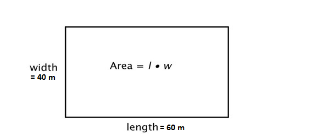QuestionAnswers

# A rectangular lawn 60 meters by 40 meters has two roads each 5 meters wide running in the middle of it one parallel to length and the other parallel to breadth. The cost of gravelling the roads at 60 paise per sq. meter isA. Rs 300B. Rs 280C. Rs 285D. Rs 250

Hint: To solve this question we need to know the basic theory related to the rectangle. As we know A rectangle is characterized by length (L) and width (W). Both length and width are different in size. So, first we will calculate the Area of the road and already mentioned that Cost of graveling the roads is 60 paise per sq. meter. Thus, we will calculate the cost of gravelling the roads.

Given,
A rectangular lawn 60 meters by 40 meters.And also, it has two roads each 5 meters wide running in the middle of it one parallel to length and the other parallel to breadth
Area of the road = $40 \times 5 + 60 \times 5 - 5 \times 5$
= $200 + 300 - 25$
= $475$
Given,
Cost of graveling the roads =60 paise per sq. meter.
$\therefore \left( {475 \times 60} \right)$ paise
= 28500 paise
= Rs.285
Therefore, the cost of gravelling the roads at 60 paise per sq. meter is Rs.285.
So, the correct answer is “Option C”.

Note: we need to remember that A Rectangle is a four sided-quadrilateral having all the internal angles to be right-angled (90o). It is to be noted that in a rectangle the opposite sides are equal in length which makes it different from a square.
For example, if one side of a rectangle is 10 cm, then the side opposite to it is also 10 cm.